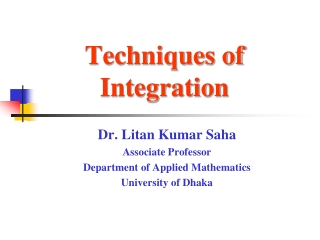DownloadDownload PresentationTechniques of Integration

# Techniques of Integration

Download Presentation## Techniques of Integration

- - - - - - - - - - - - - - - - - - - - - - - - - - - E N D - - - - - - - - - - - - - - - - - - - - - - - - - - -
##### Presentation Transcript

1. Techniques of Integration Dr. Litan Kumar Saha Associate Professor Department of Applied Mathematics University of Dhaka

2. 7.1 AN OVERVIEW OF INTEGRATION METHODS

3. TRIGONOMETRIC FUNCTIONS

4. 7.2 INTEGRATION BY PARTS First, start with the Product Rule for differentiation. This is the formula for Integration by Parts.

5. Integration by parts Let dv be the most complicated part of the original integrand that fits a basic integration Rule (including dx). Then uwill be the remaining factors. OR Let u be a portion of the integrand whose derivative is a function simpler than u. Then dvwill be the remaining factors (including dx).

6. When deciding what to choose for u, remember L I P E T. L - logarithmic function I - inverse trig function P - polynomial function E - exponential function T - trigonometry function This is usually the preference order in which you would want to choose u.

7. Integration by parts u = x dv = exdx du = dx v = ex

8. Integration by parts u = lnxdv= x2dx du = 1/x dx v = x3 /3

9. Integration by parts u = x2 dv = sin x dx du = 2x dx v = -cos x u = 2xdv = cos x dx du = 2dx v = sin x

10. Example 4 Notice that when we choose u and dv the second time, u the second time is du from the first, and dv this time is v from the first time.

11. If we were to use Integration by Parts on the last integral we would have: u = 2 dv = -cos x dx du = 0 v = -sin x Again u this time is du from the previous integral, and dv this time is v from the previous integral. First of all, finishing this integral we have: Keep this answer handy and let’s take a different look at this problem.

12. We’ll choose the same u and dv as we did the first time. But this time, let’s take the derivative of u all the way through and integrate dv for everytime we differentiate u. u dv Notice that when we keep taking the derivative of u it eventually goes to 0, and dv never runs out of integrals. (+) x2 cos x ( - ) 2x sin x (+) 2 -cos x If we multiply each u by its respective v and we switch the signs each time we add each part together we get… 0 -sin x x2 sin x + 2x cos x - 2 sin x + C This method is called tabular integration. This works when the derivatives of u eventually reach 0 and the integrals of dv never end. This is much quicker than using the formula over and over again.

13. 7.2 INTEGRATION BY PARTS

14. 7.3 INTEGRATING TRIGONOMETRIC FUNCTIONS

15. 7.4 TRIGONOMETRIC SUBSTITUTIONS

16. 7.5 INTEGRATING RATIONAL FUNCTIONS BY PARTIAL FRACTIONS